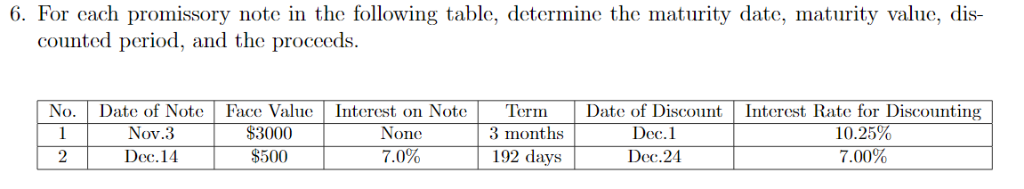Question

# Actuarial Science Mathematics of Finance Discounted Value 6. For cach promissory note in the following table, determine the maturity date, maturity value, dis- counted period, and the proceeds. No.Da...Actuarial Science Mathematics of Finance Discounted Value

6. For cach promissory note in the following table, determine the maturity date, maturity value, dis- counted period, and the proceeds. No.Date of Note Face ValueInterest on NoteTermDate of Discount Interest Rate for Discounting Nov.3 \$3000 None Dec.1 3 months \$500 Dec.24 192 days 7.00% 2 Dec.14 7.0%

No 1 -

Maturity date - February 3rd,

Maturity Value - \$3000

Discounted Period - 64 days

Proceeds - \$3000 - \$53.92 (3000 * 10.25% * 64/365) = \$2946.08

No 2 -

Maturity date - June 23rd

Maturity Value - \$518.41 (\$500 + \$18.41 (500 * 7% * 192/365))

Discounted Period - 182 days

Proceeds - \$518.41 - \$18.09 (\$518.41 * 7% * 182/365)

Note - Total no of days for year considered as 365 days

#### Earn Coins

Coins can be redeemed for fabulous gifts.

Similar Homework Help Questions
• ### Use ordinary interest: Principal \$70,000 Rate of Interest: 11% Time: 90 days Maturity Value: A Date note made Mar 10 Date note discounted: April 15 discount period: B proceeds: C note to be discounted at 10%

Use ordinary interest: Principal \$70,000 Rate of Interest: 11% Time: 90 days Maturity Value: A Date note made Mar 10 Date note discounted: April 15 discount period: B proceeds: C note to be discounted at 10%